###### back to index | new

A block of wood has the shape of a right circular cylinder with radius $6$ and height $8$, and its entire surface has been painted blue. Points $A$ and $B$ are chosen on the edge of one of the circular faces of the cylinder so that $\overset\frown{AB}$ on that face measures $120^\text{o}$. The block is then sliced in half along the plane that passes through point $A$, point $B$, and the center of the cylinder, revealing a flat, unpainted face on each half. The area of one of these unpainted faces is $a\cdot\pi + b\sqrt{c}$, where $a$, $b$, and $c$ are integers and $c$ is not divisible by the square of any prime. Find $a+b+c$.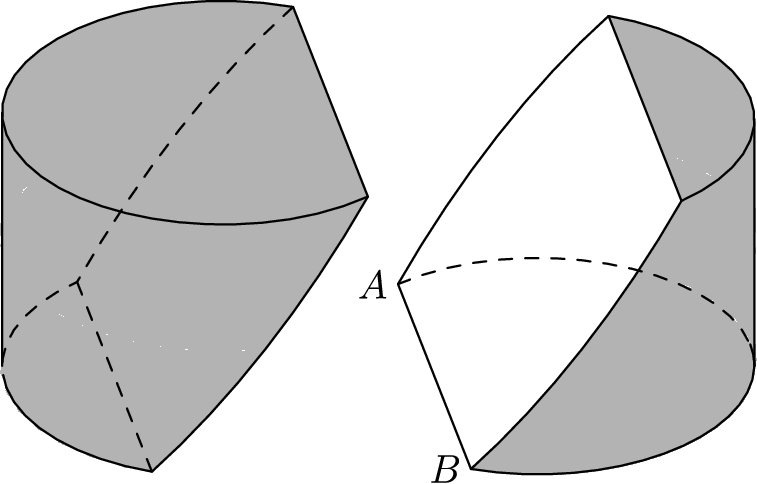In $\triangle BAC$, $\angle BAC=40^\circ$, $AB=10$, and $AC=6$. Points $D$ and $E$ lie on $\overline{AB}$ and $\overline{AC}$ respectively. What is the minimum possible value of $BE+DE+CD$?

$ABCD$ is a square of side length $\sqrt{3} + 1$. Point $P$ is on $\overline{AC}$ such that $AP = \sqrt{2}$. The square region bounded by $ABCD$ is rotated $90^{\circ}$ counterclockwise with center $P$, sweeping out a region whose area is $\frac{1}{c} (a \pi + b)$, where $a$, $b$, and $c$ are positive integers and $\text{gcd}(a,b,c) = 1$. What is $a + b + c$?

The letter F shown below is rotated $90^\circ$ clockwise around the origin, then reflected in the $y$-axis, and then rotated a half turn around the origin. What is the final image?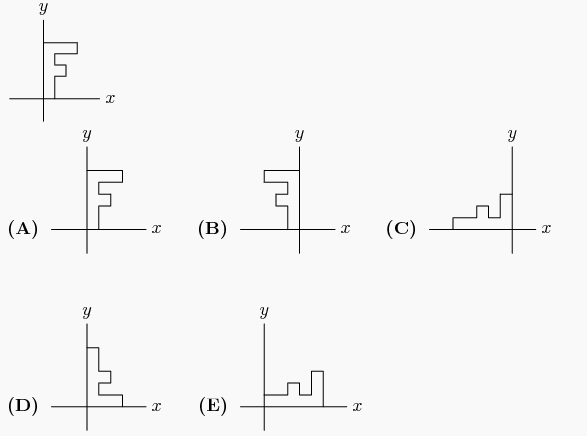A triangle with vertices $(6, 5)$, $(8, -3)$, and $(9, 1)$ is reflected about the line $x=8$ to create a second triangle. What is the area of the union of the two triangles?

Let points $A$ = $(0 ,0 ,0)$, $B$ = $(1, 0, 0)$, $C$ = $(0, 2, 0)$, and $D$ = $(0, 0, 3)$. Points $E$, $F$, $G$, and $H$ are midpoints of line segments $\overline{BD},\text{ } \overline{AB}, \text{ } \overline {AC},$ and $\overline{DC}$ respectively. What is the area of $EFGH$?

Triangle $OAB$ has $O=(0,0)$, $B=(5,0)$, and $A$ in the first quadrant. In addition, $\angle ABO=90^\circ$ and $\angle AOB=30^\circ$. Suppose that $OA$ is rotated $90^\circ$ counterclockwise about $O$. What are the coordinates of the image of $A$?

In rectangle $ABCD$, we have $A=(6,-22)$, $B=(2006,178)$, $D=(8,y)$, for some integer $y$. What is the area of rectangle $ABCD$?

Consider triangle ABC and its circumcircle S. Reflect the circle with respect to AB, AC, BC to get three new circles SAB, SBC, and SBC. Show that these three circles intersect at a common point and identify this point.

Given point $P_0$ in the plane of triangle $A_1A_2A_3$. Denote $A_s = A_{s-3}$, for $s > 3$. Construct points $P_1; P_2; \cdots$ sequentially such that point $P_{k+1}$ is $P_k$ rotated $120^\circ$ counter-clockwise around $A_{k+1}$. Prove that if $P_{1986} = P_0$ then triangle $A_1A_2A_3$ is isosceles.

Suppose the point $F$ is inside a square $ABCD$ such that $BF=1$, $FA=2$, and $FD=3$, as shown. Find the measurement of $\angle{BFA}$.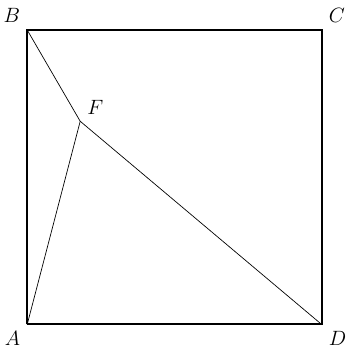Let $AD$ be the altitude in $\triangle{ABC}$ from the vertex $A$. If $\angle{A}=45^\circ$, $BD=3$, $DC=2$, find the area of $\triangle{ABC}$.

What are the coordinates of the reflection of (6,0) across the graph of $y=3x$?

A parabola with equation $y=ax^2+bx+c$ is reflected about the $x$-axis. The parabola and its reflection are translated horizontally five units in opposite directions to become the graphs of $y=f(x)$ and $y=g(x)$, respectively. Which of the following describes the graph of $y=(f+g)(x)$?

A triangle with vertices $A(0, 2)$, $B(-3, 2)$, and $C(-3, 0)$ is reflected about the $x$-axis, then the image $\triangle A'B'C'$ is rotated counterclockwise about the origin by $90^{\circ}$ to produce $\triangle A''B''C''$. Which of the following transformations will return $\triangle A''B''C''$ to $\triangle ABC$?

A dilatation of the plane—that is, a size transformation with a positive scale factor—sends the circle of radius $2$ centered at $A(2,2)$ to the circle of radius $3$ centered at $A’(5,6)$. What distance does the origin $O(0,0)$, move under this transformation?

In isosceles right triangle $\triangle{ABC}$, $\angle{C}$ is the right angle. Points $D$ and $E$ are on side $AB$ such that $\angle{DCE}=45^\circ$, $AD=25$, and $EB=16$. Let the length of $DE$ be $x$. Find $x^2$.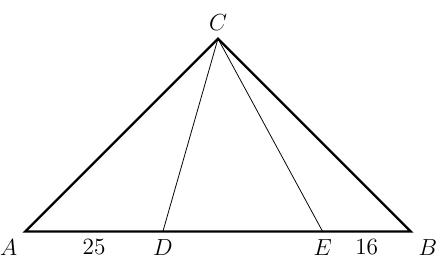Let $ABCD$ be a square. Points $E$ and $F$ are on its sides $BC$ and $CD$ such that $\angle{EAF}=45^\circ$. If point $G$ is on $EF$ such that $AG\perp EF$, show $AG=AD$.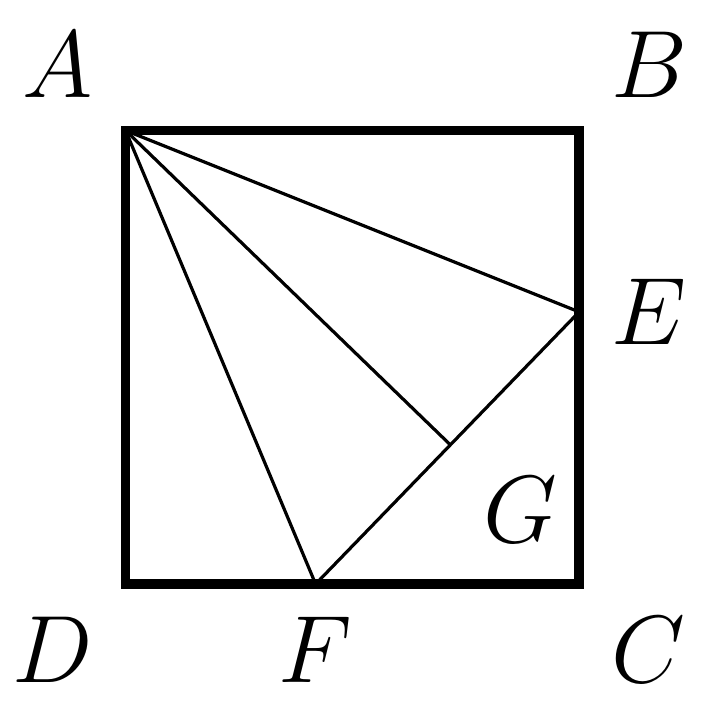Let $D$ be a point inside an isosceles triangle $\triangle{ABC}$ where $AB=AC$. If $\angle{ADB} > \angle{ADC}$, prove $DC > DB$.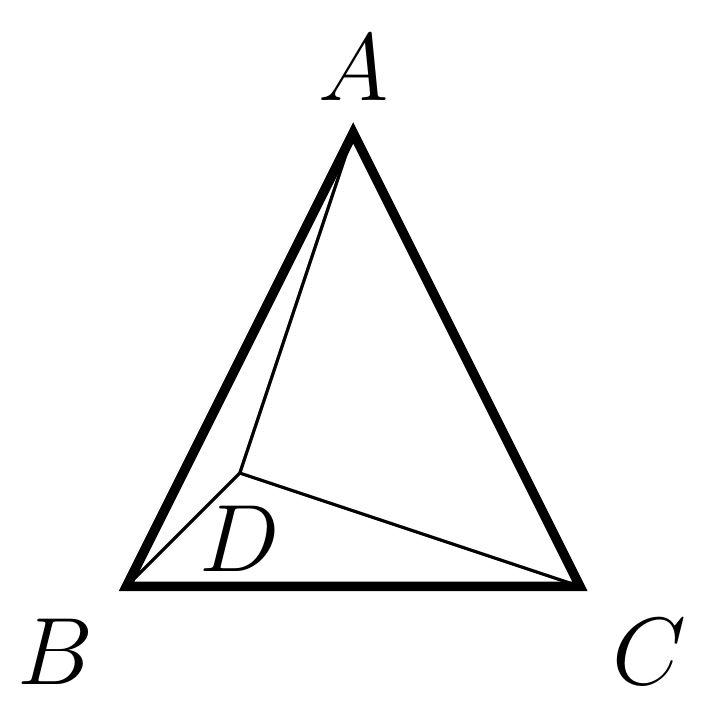Let $ABCD$ be a square. Points $E$ and $F$ are on side $CD$ and $BC$, respectively such that $AF$ bisects $\angle{EAB}$. Show that $AE=BE+DF$.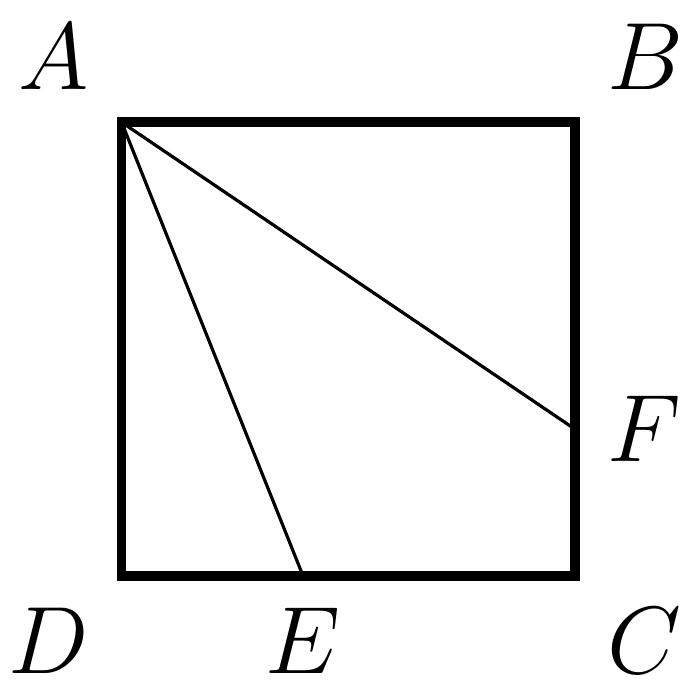Let $ABCD$ be a square with side length of 1. Find the total area of the shaded parts in the diagram.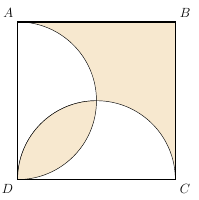As shown, in quadrilateral $ABCD$, $AB=AD$, $\angle{BAD} = \angle{DCB} = 90^\circ$. Draw altitude from $A$ towards $BC$ and let the foot be $E$. If $AE=1$, find the area of $ABCD$.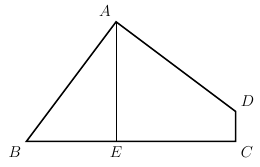In pentagon $ABCDE$, if $AB=AE$, $BC+DE=CD$, and $\angle{ABC} + \angle{AED} = 180^\circ$, show that $\angle{ADE}=\angle{ADC}$.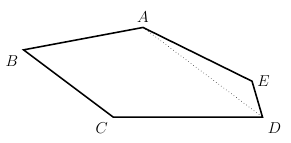Let point $P$ be inside equilateral $\triangle{ABC}$ such that $PA=2$, $PB=2\sqrt{3}$, and $PC=4$. Find the side length of this equilateral triangle.

Let point $P$ be inside of equilateral $\triangle{ABC}$ such that $PA=3, PB=4,$ and $PC=5$. Find the measurement of $\angle{APB}$.